# Tutorial: Using pyMOR’s discretization toolkit¶

pyMOR’s discretization toolkit allows to quickly build parameterized full-order models based on the NumPy/SciPy software stack. Currently supported are stationary or instationary scalar PDEs of up to second order with possibly nonlinear advection or reaction terms in one or two spatial dimensions. Unstructured meshes can be imported in the Gmsh file format.

In this tutorial we will focus on elliptic equations of the form

$-\nabla \cdot \big(\sigma(x, \mu) \nabla u(x, \mu) \big) = f(x, \mu),\quad x \in \Omega,$

on the domain $$\Omega:= (0, 1)^2 \subset \mathbb{R}^2$$ with data functions $$f(\cdot, \mu) \in L^2(\Omega)$$, $$\sigma(\cdot, \mu) \in L^\infty(\Omega)$$.

## A first equation without parameters¶

First, let us assume that the source $$f(x, \mu)$$ is an indicator function of a circular disk with radius $$0.3$$ and that $$\sigma(x, \mu)$$ is constant:

$\begin{split}f(x, \mu) := \begin{cases} 1, & |x - (0.5, 0.5)| < 0.3, \\ 0, & \text{otherwise}, \end{cases} \quad\text{and}\quad \sigma(x, \mu) :\equiv 1.\end{split}$

We start by importing commonly used pyMOR classes and methods from the basic module:

from pymor.basic import *


To specify the problem at hand using pyMOR’s discretization toolkit, we first need to specify the computational domain $$\Omega$$. Multiple classes are available to define such domains in the domaindescriptions module, which all derive from the DomainDescription interface class.

In our case, we can use a RectDomain:

domain = RectDomain([[0.,0.], [1.,1.]])


Data functions are defined using classes which derive from the Function interface. We specify the constant diffusivity $$\sigma$$ using a ConstantFunction:

diffusion = ConstantFunction(1, 2)


Here, the first argument is the function’s constant value. The second argument is the spatial dimension of the domain the problem is defined on.

For the definition of the source term $$f$$ we use an ExpressionFunction which is given an arbitrary Python expression used to evaluate the function. In this expression, the coordinates at which the function shall be evaluated are given as the variable x. Many NumPy functions can be used directly. The entire NumPy module is available under the name np.

Thus, to define $$f$$ we could write

(sqrt((x-0.5)**2 + (x-0.5)**2) <= 0.3) * 1.


However, pyMOR Functions are required to be vectorized with respect to the coordinate x. In the case of ExpressionFunction this means that x can be an arbitrary dimensional NumPy array of coordinates where the last array index specifies the spacial dimension. Therefore, the correct definition of $$f$$ is:

rhs = ExpressionFunction('(sqrt( (x[...,0]-0.5)**2 + (x[...,1]-0.5)**2) <= 0.3) * 1.', 2, ())


Similarly to ConstantFunction, the second argument is the dimension of the computational domain. As the shape of the return value cannot be easily inferred from the given string expression, it has to be provided as a third argument to ConstantFunction. For scalar functions we provide the empty tuple (), for functions returning three-dimensional vectors we would specify (3,), and for functions returning $$2\times 2$$ matrices we would specify (2,2).

Finally, the computational domain and all data functions are collected in a StationaryProblem:

problem = StationaryProblem(
domain=domain,
diffusion=diffusion,
rhs=rhs,
)


This problem definition can now be handed over to discretization methods (see pymor.discretizers.builtin) which assemble corresponding discrete full-order models. For finite elements, we use discretize_stationary_cg, which receives the maximum mesh element diameter via the diameter argument:

m, data = discretize_stationary_cg(problem, diameter=1/4)


The resulting Model can be solved, returning a VectorArray with the solution data:

U = m.solve()


Finally, we visualize the solution:

m.visualize(U)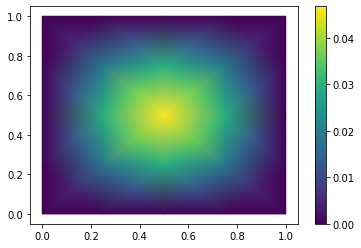In case a specific grid type shall be used (RectGrid or TriaGrid), the corresponding class has to be passed to the discretizer as the grid_type argument. By using RectGrid we get bilinear finite elements:

m, data = discretize_stationary_cg(problem, diameter=1/4, grid_type=RectGrid)
m.visualize(m.solve())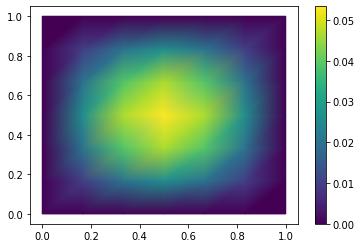We get a finite volume model using discretize_stationary_fv:

m, data = discretize_stationary_fv(problem, diameter=1/4, grid_type=TriaGrid)
m.visualize(m.solve())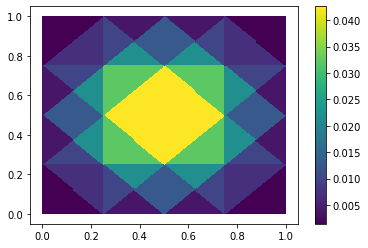## Defining boundary conditions¶

As the vigilant reader will already have noticed, we did not specify any boundary conditions when defining and solving our problem. When no boundary conditions are specified, pyMOR’s discretization toolkit will assume that homogeneous Dirichlet conditions are implied over the entire boundary of $$\Omega$$.

As the next example, let us now assume that the data functions are given by

$\begin{split}f(x, \mu) :\equiv 0 \quad\text{and}\quad \sigma(x, \mu) := \begin{cases} 0.001, & |x - (0.5, 0.5)| < 0.3, \\ 1, & \text{otherwise}, \end{cases}\end{split}$

and that we have the following mixed boundary conditions

\begin{split}\begin{align} -\sigma(x, \mu) \nabla u(x, \mu) \cdot n &= g_N(x), && x \in (0,1) \times \{0\} =: \Omega_N \\ u(x, \mu) &= 0, && x \in \partial\Omega \setminus \Omega_N, \end{align}\end{split}

with $$g_N(x) \equiv -1$$.

Before solving this problem, let us first silence pyMOR’s verbose log messages for the rest of this tutorial using the set_log_levels method:

set_log_levels({'pymor': 'WARN'})


To impose the correct boundary conditions we need to declare which type of boundary condition should be active on which part of $$\partial\Omega$$ when defining the computational domain:

domain = RectDomain(bottom='neumann')


Then all we need is to pass the Neumann data function $$g_N$$ to the StationaryProblem. Here, we can use again a ConstantFunction. The diffusivity can be defined similarly as above:

neumann_data = ConstantFunction(-1., 2)

diffusion = ExpressionFunction('1. - (sqrt( (x[...,0]-0.5)**2 + (x[...,1]-0.5)**2) <= 0.3) * 0.999' , 2, ())

problem = StationaryProblem(
domain=domain,
diffusion=diffusion,
neumann_data=neumann_data
)


Finally, we discretize and solve:

m, data = discretize_stationary_cg(problem, diameter=1/32)
m.visualize(m.solve())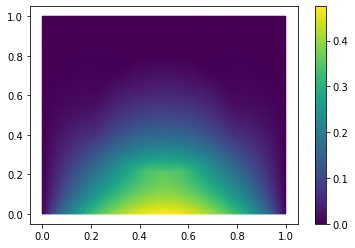## Another example¶

Even with a single ExpressionFunction we can build many different examples. For instance, to let $$\sigma$$ be given by a periodic pattern of $$K\times K$$ circular disks of radius $$0.3/K$$ we can use the following definition:

diffusion = ExpressionFunction(
'1. - (sqrt( (np.mod(x[...,0],1./K)-0.5/K)**2 + (np.mod(x[...,1],1./K)-0.5/K)**2) <= 0.3/K) * 0.999',
2, (),
values={'K': 10}
)


Here, we have used the values parameter of ExpressionFunction to make K available as an additional constant in the defining expression. In particular, we can easily change K programatically without having to resort to string manipulations. The solution looks like this:

problem = StationaryProblem(
domain=domain,
diffusion=diffusion,
neumann_data=neumann_data
)

m, data = discretize_stationary_cg(problem, diameter=1/100)
m.visualize(m.solve())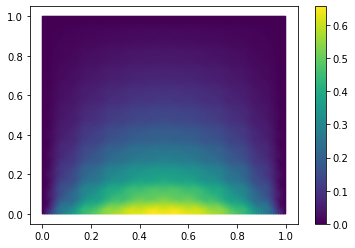## Data functions defined from pixel graphics¶

BitmapFunction uses the Python Imaging Library (PIL) to read gray scale images in various image file formats. The resulting two-dimensional NumPy array of pixel values defines a piecewise constant data function on a rectangular domain, where the range of the function (from black to white) is specified via the range parameter. For instance, when using a BitmapFunction for $$\sigma$$ with the following graphic stored in RB.png:and a range of [0.001 1] we obtain:

diffusion = BitmapFunction('RB.png', range=[0.001, 1])
problem = StationaryProblem(
domain=domain,
diffusion=diffusion,
neumann_data=neumann_data
)

m, data = discretize_stationary_cg(problem, diameter=1/100)
m.visualize(m.solve())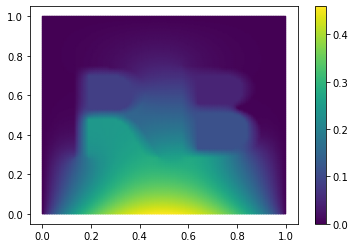The displayed warning results from the fact that the used image file has an additional channel of transparency values (alpha channel) and can be ignored.

## A parametric problem¶

Now, let us consider the Neumann data function:

$g_N((x_0, x_1), \mu_{neum}) := -\cos(\pi \cdot x_0)^2 \cdot\mu_{neum}$

with a single Parameter $$\mu_{neum} \in \mathbb{R}$$.

In pyMOR, parameter values are specified as a dictionary of one-dimensional NumPy arrays. Each value in the dictionary must have a correct size specified by the Parameters of the ParametricObject. In this example we have a single scalar valued parameter which we call 'neum'. Thus, the Parameters of the function will be

{'neum': 1}


We can then make the following definition of the Neumann data:

neumann_data = ExpressionFunction('-cos(pi*x[...,0])**2*neum', 2, (), parameters= {'neum': 1})


Similar to the range of the function, pyMOR cannot infer from the given string expression the parameters used in the expression, so these Parameters have to be provided as the parameters argument. The individual parameters are then available as variables in the expression.

We can then proceed as usual and automatically obtain a parametric Model:

diffusion = ExpressionFunction(
'1. - (sqrt( (np.mod(x[...,0],1./K)-0.5/K)**2 + (np.mod(x[...,1],1./K)-0.5/K)**2) <= 0.3/K) * 0.999',
2, (),
values={'K': 10}
)
problem = StationaryProblem(
domain=domain,
diffusion=diffusion,
neumann_data=neumann_data
)

m, data = discretize_stationary_cg(problem, diameter=1/100)
m.parameters

Parameters({neum: 1})


When solving the model, we now need to specify appropriate parameter values:

m.visualize(m.solve({'neum': [1.]}))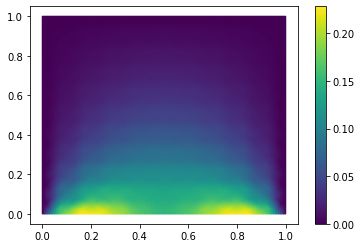For the solve method, the parameter value can also be specified as a single number:

m.visualize(m.solve(-100))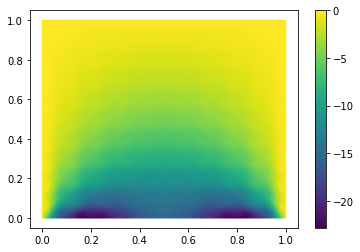## Multiple parameters¶

Next we also want to parameterize the diffusivity in the $$K \times K$$ circular disks by a scalar factor $$\mu_{diffu}$$. To this end we define:

diffusion = ExpressionFunction(
'1. - (sqrt( (np.mod(x[...,0],1./K)-0.5/K)**2 + (np.mod(x[...,1],1./K)-0.5/K)**2) <= 0.3/K) * (1 - diffu)',
2, (),
values={'K': 10},
parameters= {'diffu': 1}
)


We proceed as usual:

problem = StationaryProblem(
domain=domain,
diffusion=diffusion,
neumann_data=neumann_data
)

m, data = discretize_stationary_cg(problem, diameter=1/100)
m.parameters

Parameters({diffu: 1, neum: 1})


As we can see, pyMOR automatically derives that in this case the model depends on two Parameters, and we have to provide two values when solving the model:

m.visualize(m.solve({'diffu': 0.001, 'neum': 1}))For solve we can also simply pass a list of parameter values, in which case pyMOR assumes an alphabetical ordering of the parameters:

m.visualize(m.solve([1, -1]))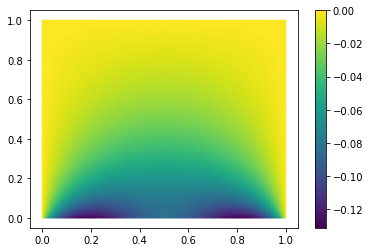## Parameter-separability¶

For the generation of online-efficient reduced-order models, it is often crucial that data functions are parameter separable. We call a parametric function f(x, mu) parameter separable if it admits a decomposition

$f(x, \mu) = \sum_{q=1}^Q f_q(x) \cdot \theta_q(\mu)$

where the $$f_q$$ are non-parametric and the parameter functionals $$\theta_q$$ map parameters to real numbers.

To model such a decomposition using pyMOR’s discretization toolkit, we specify the $$f_q$$ as non-parametric Functions, the $$\theta_q$$ are represented by ParameterFunctionals and the given sum decomposition is represented by a LincombFunction of these objects.

As an example let us go back to the case where the diffusivity is represented by indicator functions of point sets forming the letters RB. We now want to parameterize the diffusivity in the individual letters. This admits a decomposition of the form

$\sigma(x,y) = 1 + f_R \cdot (\mu_R - 1) + f_B \cdot (\mu_L - 1)$

Again, we define $$f_R$$ and $$f_L$$ as BitmapFunctions for the following image files: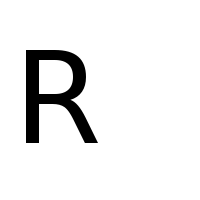f_R = BitmapFunction('R.png', range=[1, 0])
f_B = BitmapFunction('B.png', range=[1, 0])


Next we need to define the ParameterFunctionals

$\theta_R(\mu) = \mu_R - 1 \quad\text{and}\quad \theta_B(\mu) = \mu_B - 1.$

Similar to an ExpressionFunction, we can use ExpressionParameterFunctionals for that:

theta_R = ExpressionParameterFunctional('R - 1', {'R': 1})
theta_B = ExpressionParameterFunctional('B - 1', {'B': 1})


Note that the second argument is again the Parameters that are used in the expression. Finally, we form the linear combination using a LincombFunction which is given a list of Functions as the first and a corresponding list of ParameterFunctionals or constants as the second argument:

diffusion = LincombFunction(
[ConstantFunction(1., 2), f_R, f_B],
[1., theta_R, theta_B]
)
diffusion.parameters

Parameters({B: 1, R: 1})


Again, pyMOR automatically derives that the evaluation of diffusion depends on the two Parameters 'B' and 'R'. Now, we can proceed as usual:

problem = StationaryProblem(
domain=domain,
diffusion=diffusion,
neumann_data=ConstantFunction(-1, 2)
)
m, data = discretize_stationary_cg(problem, diameter=1/100)
m.visualize((m.solve([1., 0.001]), m.solve([0.001, 1])))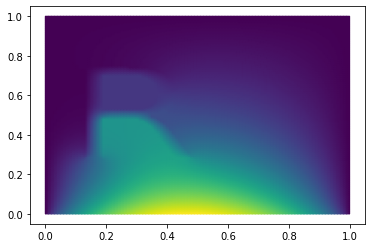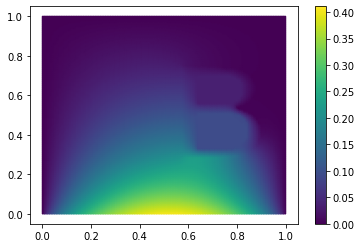Looking at the Model m, we can see that the decomposition of $$\sigma$$ has been preserved by the discretizer:

m.operator

LincombOperator(
(NumpyMatrixOperator(<20201x20201 sparse, 140601 nnz>, source_id='STATE', range_id='STATE', name='boundary_part'),
NumpyMatrixOperator(<20201x20201 sparse, 140601 nnz>, source_id='STATE', range_id='STATE', name='diffusion_0'),
NumpyMatrixOperator(<20201x20201 sparse, 140601 nnz>, source_id='STATE', range_id='STATE', name='diffusion_1'),
NumpyMatrixOperator(<20201x20201 sparse, 140601 nnz>, source_id='STATE', range_id='STATE', name='diffusion_2')),
(1.0, 1.0, ExpressionParameterFunctional('R - 1', {R: 1}), ExpressionParameterFunctional('B - 1', {B: 1})),
name='ellipticOperator')


The LincombFunction has become a LincombOperator, with the same linear coefficients but the BitmapFunctions replaced by corresponding stiffness matrices. Note that an additional summand appears which ensures correct enforcement of Dirichlet boundary values for all possible parameter value combinations.